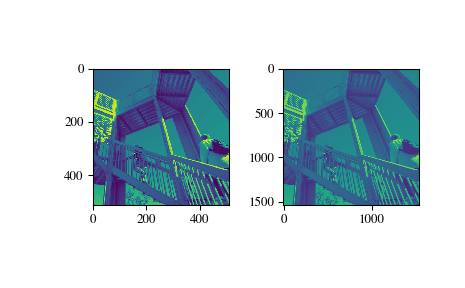# scipy.ndimage.zoom¶

scipy.ndimage.zoom(input, zoom, output=None, order=3, mode='constant', cval=0.0, prefilter=True)[source]

Zoom an array.

The array is zoomed using spline interpolation of the requested order.

Parameters: input : array_like The input array. zoom : float or sequence The zoom factor along the axes. If a float, zoom is the same for each axis. If a sequence, zoom should contain one value for each axis. output : array or dtype, optional The array in which to place the output, or the dtype of the returned array. By default an array of the same dtype as input will be created. order : int, optional The order of the spline interpolation, default is 3. The order has to be in the range 0-5. mode : {‘reflect’, ‘constant’, ‘nearest’, ‘mirror’, ‘wrap’}, optional The mode parameter determines how the input array is extended when the filter overlaps a border. Default is ‘reflect’. Behavior for each valid value is as follows: ‘reflect’ (d c b a | a b c d | d c b a) The input is extended by reflecting about the edge of the last pixel. ‘constant’ (k k k k | a b c d | k k k k) The input is extended by filling all values beyond the edge with the same constant value, defined by the cval parameter. ‘nearest’ (a a a a | a b c d | d d d d) The input is extended by replicating the last pixel. ‘mirror’ (d c b | a b c d | c b a) The input is extended by reflecting about the center of the last pixel. ‘wrap’ (a b c d | a b c d | a b c d) The input is extended by wrapping around to the opposite edge. cval : scalar, optional Value to fill past edges of input if mode is ‘constant’. Default is 0.0. prefilter : bool, optional Determines if the input array is prefiltered with spline_filter before interpolation. The default is True, which will create a temporary float64 array of filtered values if order > 1. If setting this to False, the output will be slightly blurred if order > 1, unless the input is prefiltered, i.e. it is the result of calling spline_filter on the original input. zoom : ndarray The zoomed input.

Examples

>>> from scipy import ndimage, misc
>>> import matplotlib.pyplot as plt

>>> fig = plt.figure()
>>> ax1 = fig.add_subplot(121)  # left side
>>> ax2 = fig.add_subplot(122)  # right side
>>> ascent = misc.ascent()
>>> result = ndimage.zoom(ascent, 3.0)
>>> ax1.imshow(ascent)
>>> ax2.imshow(result)
>>> plt.show()>>> print(ascent.shape)
(512, 512)

>>> print(result.shape)
(1536, 1536)


#### Previous topic

scipy.ndimage.spline_filter1d

#### Next topic

scipy.ndimage.center_of_mass